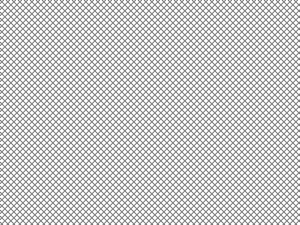Open in App
Not now

# Wand Pseudo Image – Python

• Last Updated : 24 Feb, 2023

Wand supports a number of image format specification that is some image that are created with some algorithms. Pseudo image is very important we can perform various manipulations to the image using Pseudo Image in wand. We can create pseudo image using setting pseudo parameter in Image() function.

Some manipulations we can perform – 1. Create solid color image. 2. Create gradient images. 3. Create images with patterns 4. Creates a fractal image.

Some important pseudo images :

Syntax :

## Python3

 `with Image(width``=``image_width, height``=``image_height,``                     ``pseudo``=``'pseudo_type'``) as img:``    ``# other manipulation code``   `

Now let’s get forward towards the python code for pseudo image. Example 1: Create an image with a gradient

## Python3

 `# Import Image from wand.image module``from` `wand.image ``import` `Image` `# Create pseudo image using Image() function``with Image(width ``=` `400``, height ``=` `300``,``        ``pseudo ``=``'gradient:# 32a852-# 09e846'``) as img:` `    ``# Save image with a validfilename``    ``img.save(filename ``=``'gradient.png'``)`

Output :Example 2: Create an image with a pattern

## Python3

 `# Import Image from wand.image module``from` `wand.image ``import` `Image` `# Create image using Image() and label CROSSHATCH45 for pattern``with Image(width ``=` `100``, height ``=` `100``, pseudo ``=``'pattern:CROSSHATCH45'``) as img:` `    ``# Save image``    ``img.save(filename ``=``'pattern.png'``)`My Personal Notes arrow_drop_up